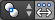# Median Point as Pivot

Mode: Object mode and Edit mode

Hotkey: Ctrl,

Menu: Select from the following icon in the 3D window headerThe Median Point can be considered to be broadly similar to the concept of Center of Gravity (COG). If we assume that every element (Object, face, vertex etc) of the selection has the same mass, the median point would sit at the point of equilibrium for the selection (the COG).

## In Object Mode

In Object Mode, Blender only considers the Object centers when determining the median point. This can lead to some counterintuitive results. In the Object Mode median points image below, you can see that the median point is between the Object centers and can be nowhere near the Objects' mesh.

## In Edit Mode

In Edit Mode, the median point is determined via the part of the selection that has the most elements. For example, in the Median points in Edit Mode image, when there are two cubes with an equal number of vertices, the median point lies directly between the two cubes. However, if we subdivide one cube multiple times so that it has many more vertices, you can see that the median point has shifted to the region with the most vertices.